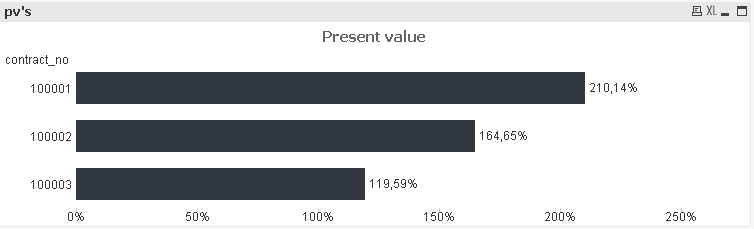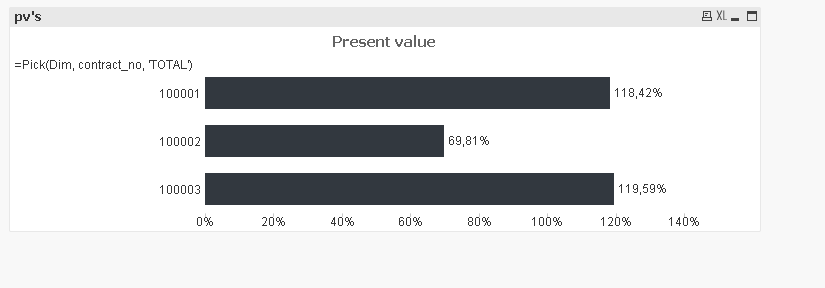# QlikView App Development

Discussion Board for collaboration related to QlikView App Development.

Announcements
Leverage your QlikView investment to modernize BI – see how! Join Group
cancel
Showing results for
Did you mean:
HighlightedCreator II

## sum over if statement with integrated set analysis function

Hello Community,

this is my database

contract_numbercontract_start_datecontract_end_datecontract_termcontract_term_typecontract_payment_perc

100001

01.01.201231.12.201436first3,11%
10000101.01.201530.06.20156second2%
10000101.07.201631.12.20166third1,5%
10000201.01.201331.12.201648first2,66%
10000201.01.201731.12.201712second1,5%
10000201.01.201830.06.20186third0,5%
10000301.01.201731.12.201936first3,05%
10000301.01.202030.06.20206second1,9%
10000301.07.202031.12.20206third1,45%

I created a variable v_target_date to calculate my present value depended on the variable v_target_date

I created in the script a new field:

lpm_history:

My expression :

if(((year(v_target_date)-year(contract_begin_ext_simulation))*12+(month(v_target_date)-month(contract_begin_ext_simulation))+1)<=0,

(PV(0.01/12, sum({<contract_term_type={'first'}>} contract_term), sum({<contract_term_type={'first'}>} contract_payment_perc),0,1)*-1),

if(((year(v_target_date)-year(contract_begin_ext_simulation))*12+(month(v_target_date)-month(contract_begin_ext_simulation))+1)>0 AND

((year(v_target_date)-year(contract_begin_ext_simulation))*12+(month(v_target_date)-month(contract_begin_ext_simulation))+1)<=sum({<contract_term_type={'second'}>} contract_term),

PV(0.01/12, sum({<contract_term_type={'first'}>} contract_term), sum({<contract_term_type={'first'}>} contract_payment_perc),0,1)*-1+

PV(0.01/12, ((year(v_target_date)-year(contract_begin_ext_simulation))*12+(month(v_target_date)-month(contract_begin_ext_simulation))+1), sum({<contract_term_type={'second'}>} contract_payment_perc),0,1)*-1,

if(((year(v_target_date)-year(contract_begin_ext_simulation))*12+(month(v_target_date)-month(contract_begin_ext_simulation))+1)>0 AND

((year(v_target_date)-year(contract_begin_ext_simulation))*12+(month(v_target_date)-month(contract_begin_ext_simulation))+1) > sum({<contract_term_type={'second'}>} contract_term),

PV(0.01/12, sum({<contract_term_type={'second'}>} contract_term), sum({<contract_term_type={'first'}>} contract_payment_perc),0,1)*-1+

PV(0.01/12, sum({<contract_term_type={'second'}>} contract_term), sum({<contract_term_type={'second'}>} contract_payment_perc),0,1)*-1+

PV(0.01/12, ((year(v_target_date)-year(contract_begin_ext_simulation))*12+(month(v_target_date)-month(contract_begin_ext_simulation))+1)-sum({<contract_term_type={'third'}>} contract_term), sum({<contract_term_type={'third'}>} contract_payment_perc),0,1)*-1

)))

i marked the set analysis in red

This is what QV returnsEverything is right so far

What I'd like to do in the next step is to take the sum of all

-> 210,14%+164,65%+119,59% = 494,38% and return the result in a textbox

unfortunetely I can't just add a sum() in front of my expression.

Is there any possibility to do that?

1 Solution

Accepted Solutions
HighlightedMVP

## Re: sum over if statement with integraded set analysis function

Can you try this....

Keep the dimension same as Anil mentioned, but change your expression to this

Sum(Aggr(if(((year(v_target_date)-year(contract_begin_ext_simulation))*12+(month(v_target_date)-month(contract_begin_ext_simulation))+1)<=0,

(PV(0.01/12, sum({<contract_term_type={'first'}>} contract_term), sum({<contract_term_type={'first'}>} contract_payment_perc),0,1)*-1),

if(((year(v_target_date)-year(contract_begin_ext_simulation))*12+(month(v_target_date)-month(contract_begin_ext_simulation))+1)>0 AND

((year(v_target_date)-year(contract_begin_ext_simulation))*12+(month(v_target_date)-month(contract_begin_ext_simulation))+1)<=sum({<contract_term_type={'second'}>} contract_term),

PV(0.01/12, sum({<contract_term_type={'first'}>} contract_term), sum({<contract_term_type={'first'}>} contract_payment_perc),0,1)*-1+

PV(0.01/12, ((year(v_target_date)-year(contract_begin_ext_simulation))*12+(month(v_target_date)-month(contract_begin_ext_simulation))+1), sum({<contract_term_type={'second'}>} contract_payment_perc),0,1)*-1,

if(((year(v_target_date)-year(contract_begin_ext_simulation))*12+(month(v_target_date)-month(contract_begin_ext_simulation))+1)>0 AND

((year(v_target_date)-year(contract_begin_ext_simulation))*12+(month(v_target_date)-month(contract_begin_ext_simulation))+1) > sum({<contract_term_type={'second'}>} contract_term),

PV(0.01/12, sum({<contract_term_type={'second'}>} contract_term), sum({<contract_term_type={'first'}>} contract_payment_perc),0,1)*-1+

PV(0.01/12, sum({<contract_term_type={'second'}>} contract_term), sum({<contract_term_type={'second'}>}contract_payment_perc),0,1)*-1+

PV(0.01/12, ((year(v_target_date)-year(contract_begin_ext_simulation))*12+(month(v_target_date)-month(contract_begin_ext_simulation))+1)-sum({<contract_term_type={'third'}>} contract_term),sum({<contract_term_type={'third'}>} contract_payment_perc),0,1)*-1

))), Contract_Number))

6 Replies
HighlightedMVP

## Re: sum over if statement with integraded set analysis function

Create one inline table like

Dim

1

2

];

And use dimension like

Pick(Dim, contract_no, 'TOTAL')

Expression is

Pick(Dim,

if(((year(v_target_date)-year(contract_begin_ext_simulation))*12+(month(v_target_date)-month(contract_begin_ext_simulation))+1)<=0,

(PV(0.01/12, sum({<contract_term_type={'first'}>} contract_term), sum({<contract_term_type={'first'}>} contract_payment_perc),0,1)*-1),

if(((year(v_target_date)-year(contract_begin_ext_simulation))*12+(month(v_target_date)-month(contract_begin_ext_simulation))+1)>0 AND

((year(v_target_date)-year(contract_begin_ext_simulation))*12+(month(v_target_date)-month(contract_begin_ext_simulation))+1)<=sum({<contract_term_type={'second'}>} contract_term),

PV(0.01/12, sum({<contract_term_type={'first'}>} contract_term), sum({<contract_term_type={'first'}>} contract_payment_perc),0,1)*-1+

PV(0.01/12, ((year(v_target_date)-year(contract_begin_ext_simulation))*12+(month(v_target_date)-month(contract_begin_ext_simulation))+1), sum({<contract_term_type={'second'}>} contract_payment_perc),0,1)*-1,

if(((year(v_target_date)-year(contract_begin_ext_simulation))*12+(month(v_target_date)-month(contract_begin_ext_simulation))+1)>0 AND

((year(v_target_date)-year(contract_begin_ext_simulation))*12+(month(v_target_date)-month(contract_begin_ext_simulation))+1) > sum({<contract_term_type={'second'}>} contract_term),

PV(0.01/12, sum({<contract_term_type={'second'}>} contract_term), sum({<contract_term_type={'first'}>} contract_payment_perc),0,1)*-1+

PV(0.01/12, sum({<contract_term_type={'second'}>} contract_term), sum({<contract_term_type={'second'}>}contract_payment_perc),0,1)*-1+

PV(0.01/12, ((year(v_target_date)-year(contract_begin_ext_simulation))*12+(month(v_target_date)-month(contract_begin_ext_simulation))+1)-sum({<contract_term_type={'third'}>} contract_term),sum({<contract_term_type={'third'}>} contract_payment_perc),0,1)*-1

))),

if(((year(v_target_date)-year(contract_begin_ext_simulation))*12+(month(v_target_date)-month(contract_begin_ext_simulation))+1)<=0,

(PV(0.01/12, sum({<contract_term_type={'first'}>} contract_term), sum({<contract_term_type={'first'}>} TOTAL contract_payment_perc),0,1)*-1),

if(((year(v_target_date)-year(contract_begin_ext_simulation))*12+(month(v_target_date)-month(contract_begin_ext_simulation))+1)>0 AND

((year(v_target_date)-year(contract_begin_ext_simulation))*12+(month(v_target_date)-month(contract_begin_ext_simulation))+1)<=sum({<contract_term_type={'second'}>} TOTAL contract_term),

PV(0.01/12, sum({<contract_term_type={'first'}>} TOTAL contract_term), sum({<contract_term_type={'first'}>} TOTAL contract_payment_perc),0,1)*-1+

PV(0.01/12, ((year(v_target_date)-year(contract_begin_ext_simulation))*12+(month(v_target_date)-month(contract_begin_ext_simulation))+1), sum({<contract_term_type={'second'}>} TOTAL contract_payment_perc),0,1)*-1,

if(((year(v_target_date)-year(contract_begin_ext_simulation))*12+(month(v_target_date)-month(contract_begin_ext_simulation))+1)>0 AND

((year(v_target_date)-year(contract_begin_ext_simulation))*12+(month(v_target_date)-month(contract_begin_ext_simulation))+1) > sum({<contract_term_type={'second'}>} TOTAL contract_term),

PV(0.01/12, sum({<contract_term_type={'second'}>} TOTAL contract_term), sum({<contract_term_type={'first'}>} TOTAL contract_payment_perc),0,1)*-1+

PV(0.01/12, sum({<contract_term_type={'second'}>} TOTAL contract_term), sum({<contract_term_type={'second'}>} TOTAL contract_payment_perc),0,1)*-1+

PV(0.01/12, ((year(v_target_date)-year(contract_begin_ext_simulation))*12+(month(v_target_date)-month(contract_begin_ext_simulation))+1)-sum({<contract_term_type={'third'}>} TOTAL contract_term),sum({<contract_term_type={'third'}>} TOTAL contract_payment_perc),0,1)*-1

))))

Before develop something, think If placed (The Right information | To the right people | At the Right time | In the Right place | With the Right context)
HighlightedCreator II

## Re: sum over if statement with integraded set analysis function

Hello Anil , thanks for your help

I just follow ur instructions. But QV returns me thisthe result should be:

210,14%+164,65%+119,59% = 494,38% and return the result in a textbox

and could u maybe explain me what the function dim() is exactly doing?

HighlightedMVP

## Re: sum over if statement with integraded set analysis function

When you say, You expression works. That means The above may work as expected. And, I just throw a stone but no luck for you. Will you able to provide sample?

And show the image / it would be great if you share sample file

Before develop something, think If placed (The Right information | To the right people | At the Right time | In the Right place | With the Right context)
HighlightedMVP

## Re: sum over if statement with integraded set analysis function

Can you try this....

Keep the dimension same as Anil mentioned, but change your expression to this

Sum(Aggr(if(((year(v_target_date)-year(contract_begin_ext_simulation))*12+(month(v_target_date)-month(contract_begin_ext_simulation))+1)<=0,

(PV(0.01/12, sum({<contract_term_type={'first'}>} contract_term), sum({<contract_term_type={'first'}>} contract_payment_perc),0,1)*-1),

if(((year(v_target_date)-year(contract_begin_ext_simulation))*12+(month(v_target_date)-month(contract_begin_ext_simulation))+1)>0 AND

((year(v_target_date)-year(contract_begin_ext_simulation))*12+(month(v_target_date)-month(contract_begin_ext_simulation))+1)<=sum({<contract_term_type={'second'}>} contract_term),

PV(0.01/12, sum({<contract_term_type={'first'}>} contract_term), sum({<contract_term_type={'first'}>} contract_payment_perc),0,1)*-1+

PV(0.01/12, ((year(v_target_date)-year(contract_begin_ext_simulation))*12+(month(v_target_date)-month(contract_begin_ext_simulation))+1), sum({<contract_term_type={'second'}>} contract_payment_perc),0,1)*-1,

if(((year(v_target_date)-year(contract_begin_ext_simulation))*12+(month(v_target_date)-month(contract_begin_ext_simulation))+1)>0 AND

((year(v_target_date)-year(contract_begin_ext_simulation))*12+(month(v_target_date)-month(contract_begin_ext_simulation))+1) > sum({<contract_term_type={'second'}>} contract_term),

PV(0.01/12, sum({<contract_term_type={'second'}>} contract_term), sum({<contract_term_type={'first'}>} contract_payment_perc),0,1)*-1+

PV(0.01/12, sum({<contract_term_type={'second'}>} contract_term), sum({<contract_term_type={'second'}>}contract_payment_perc),0,1)*-1+

PV(0.01/12, ((year(v_target_date)-year(contract_begin_ext_simulation))*12+(month(v_target_date)-month(contract_begin_ext_simulation))+1)-sum({<contract_term_type={'third'}>} contract_term),sum({<contract_term_type={'third'}>} contract_payment_perc),0,1)*-1

))), Contract_Number))

HighlightedCreator II

## Re: sum over if statement with integraded set analysis function